# Meet the Robots That Will Make Tomorrow’s Gadgets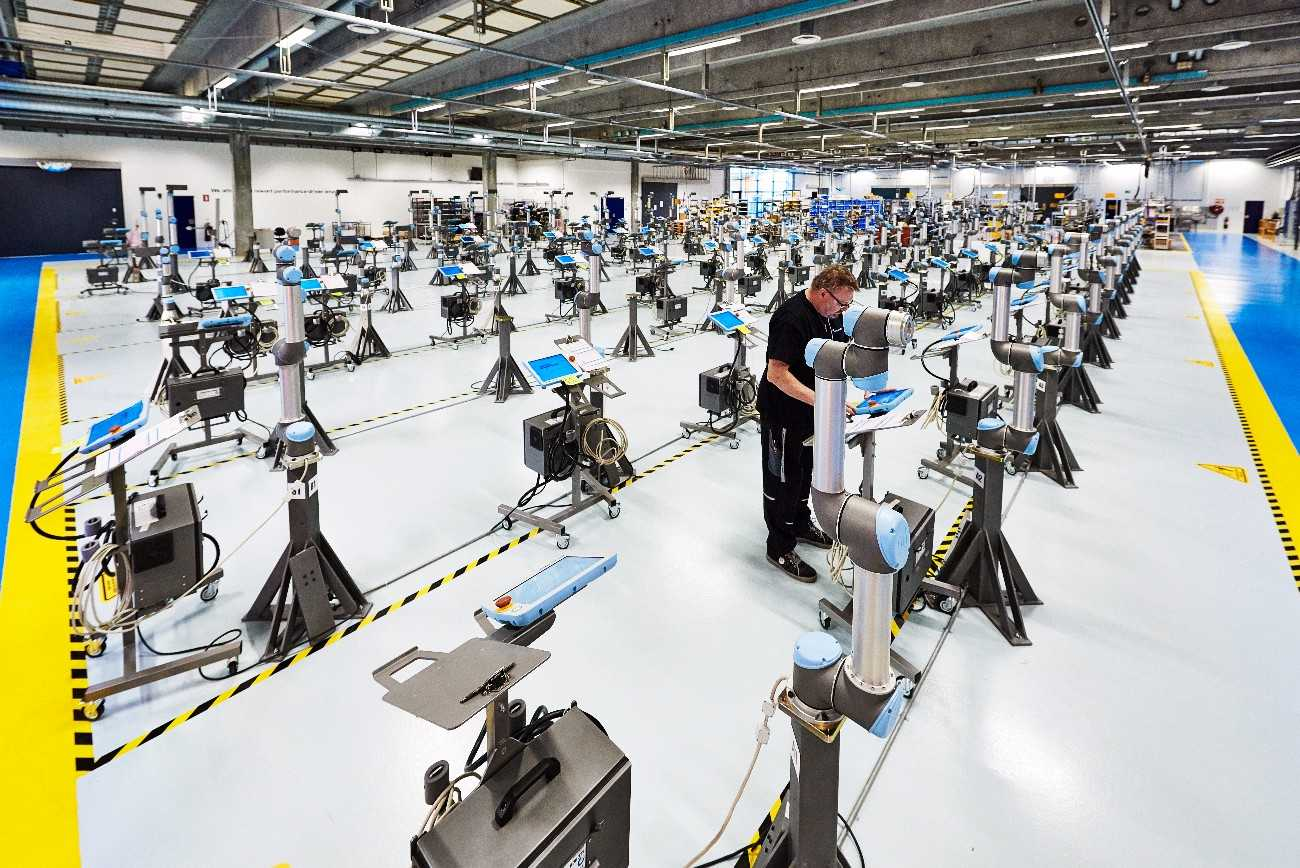From Westworld to The Terminator, movies have often imagined a dystopian future where deadly robots would cause havoc. Another popular characterization is a cute, human-like machine — think WALL-E or the Star Wars droids. Yet our modern reality is something quite different. Most robots work in factories, building the phones and cars we use every day. But what about the future? Rather than working under human supervision, machines are starting to construct things by themselves. It’s time to meet the robots that will make tomorrow’s gadgets.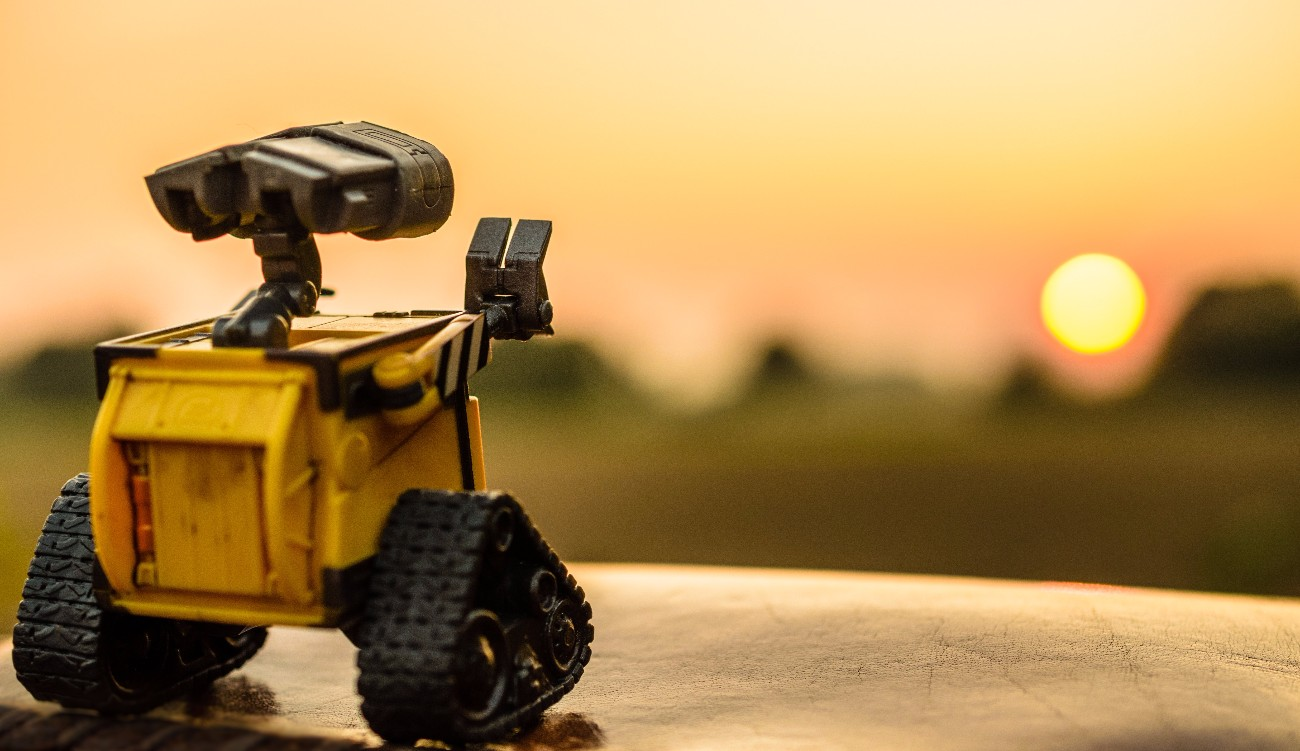## Meet the Robots

Consider for a moment how the shirt on your back came into being. The manufacturing process for even a simple garment like this is surprisingly complex.

The maker must first source the correct material, and then create or inherit a design. Next, the cloth must be cut to size, with such precision that each piece can be neatly sewn together. Then the needle must weave its path and pull the thread into a perfect join. Finally, the finished garment should be checked and packed for shipping.

While intelligent machines can handle some of these tasks, they cannot yet take over the entire process. Or can they?

A micro-factory in Brooklyn recently became the first ever humanless production line. The space looks much like a small office, except the walls are lined with rows of 3D printers rather than desks. The printers work 24 hours a day, creating custom parts and prototypes for customers around the country.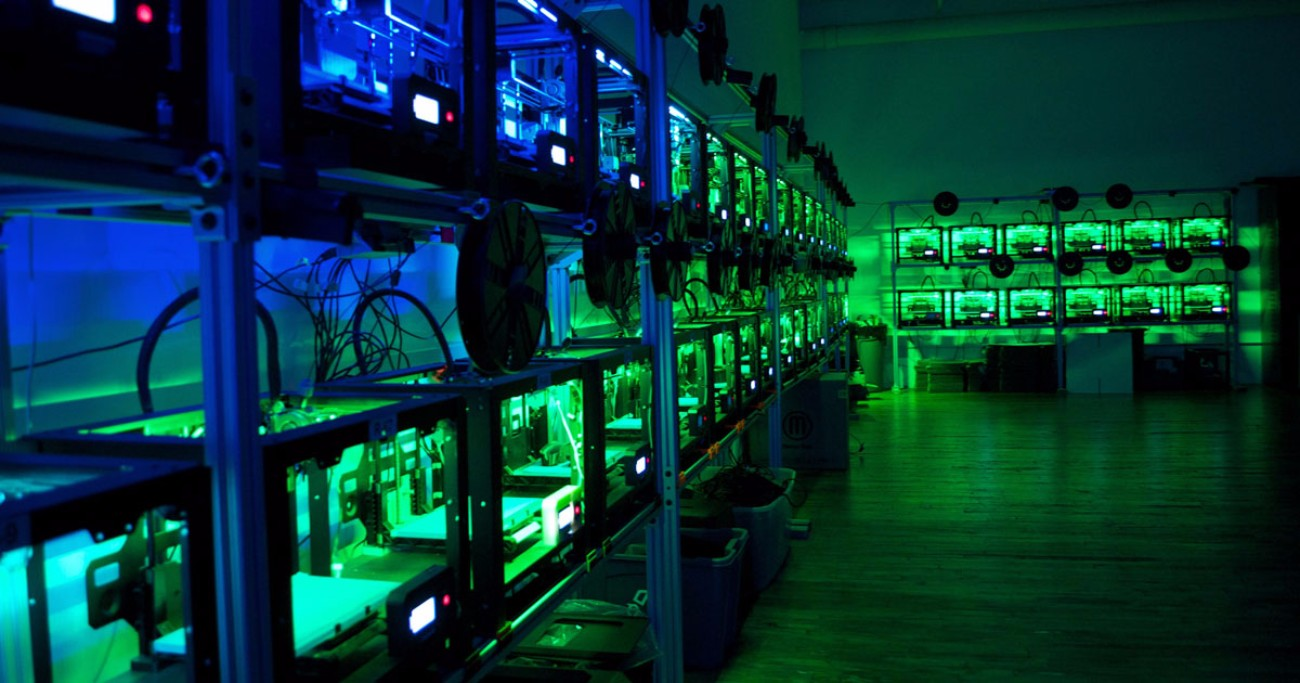For all this frenetic activity, there are very few bodies around. In fact, this hub of productivity has the ambience of a server room. That’s because VooDoo Manufacturing — the startup which runs this system — relies on robots to look after the printers.

For the time being, this factory is more of a working demo than a large-scale operation. Humans still load the spools and perform other routine tasks. But at some point, those same humans could be free to use their creativity rather than dealing with maintenance issues.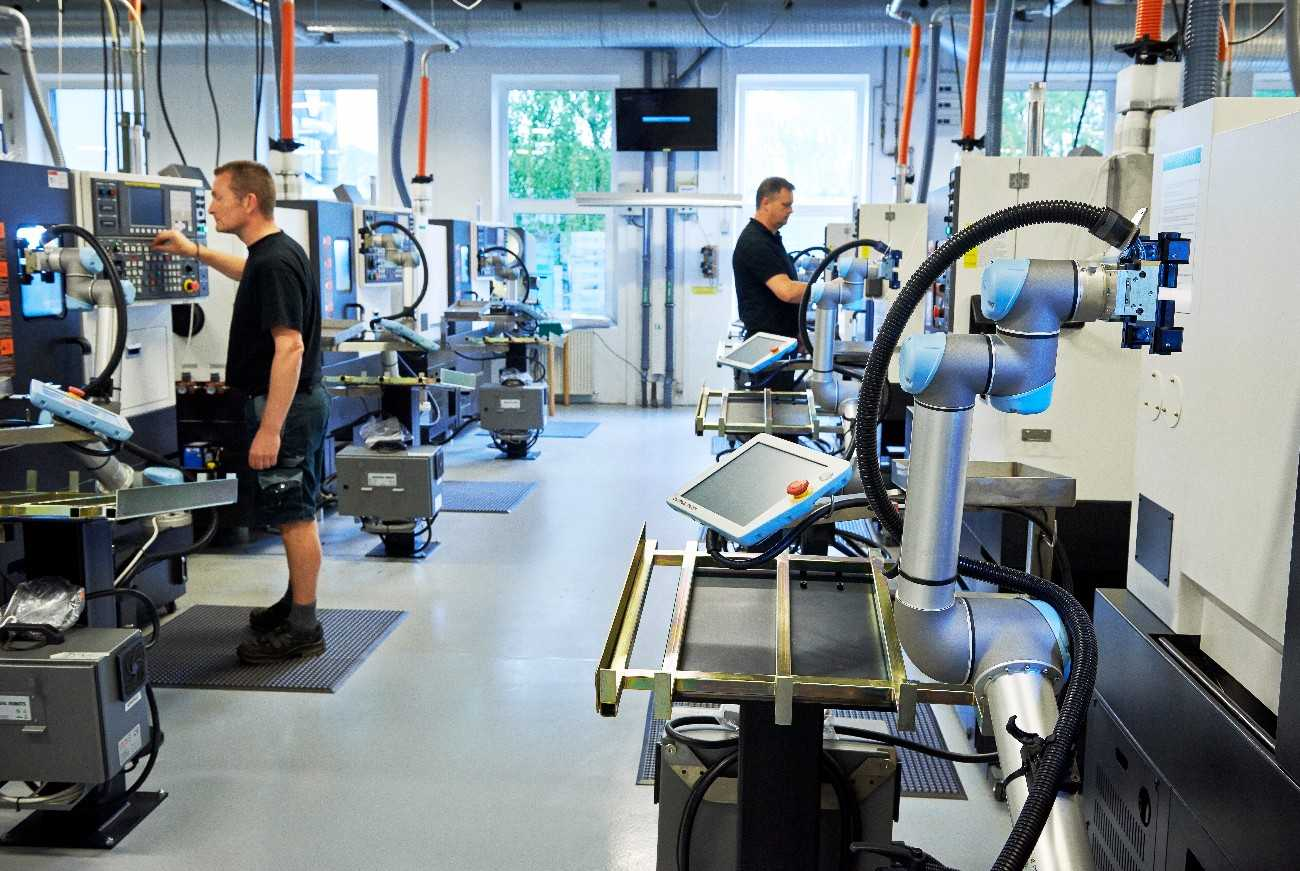## Machine-Human Collaboration

This kind of setup hints at a future where robots will replace humans on the factory floor. In some cases, this will probably happen. But many industry insiders believe that these machines are merely tools for us to use, rather than competition.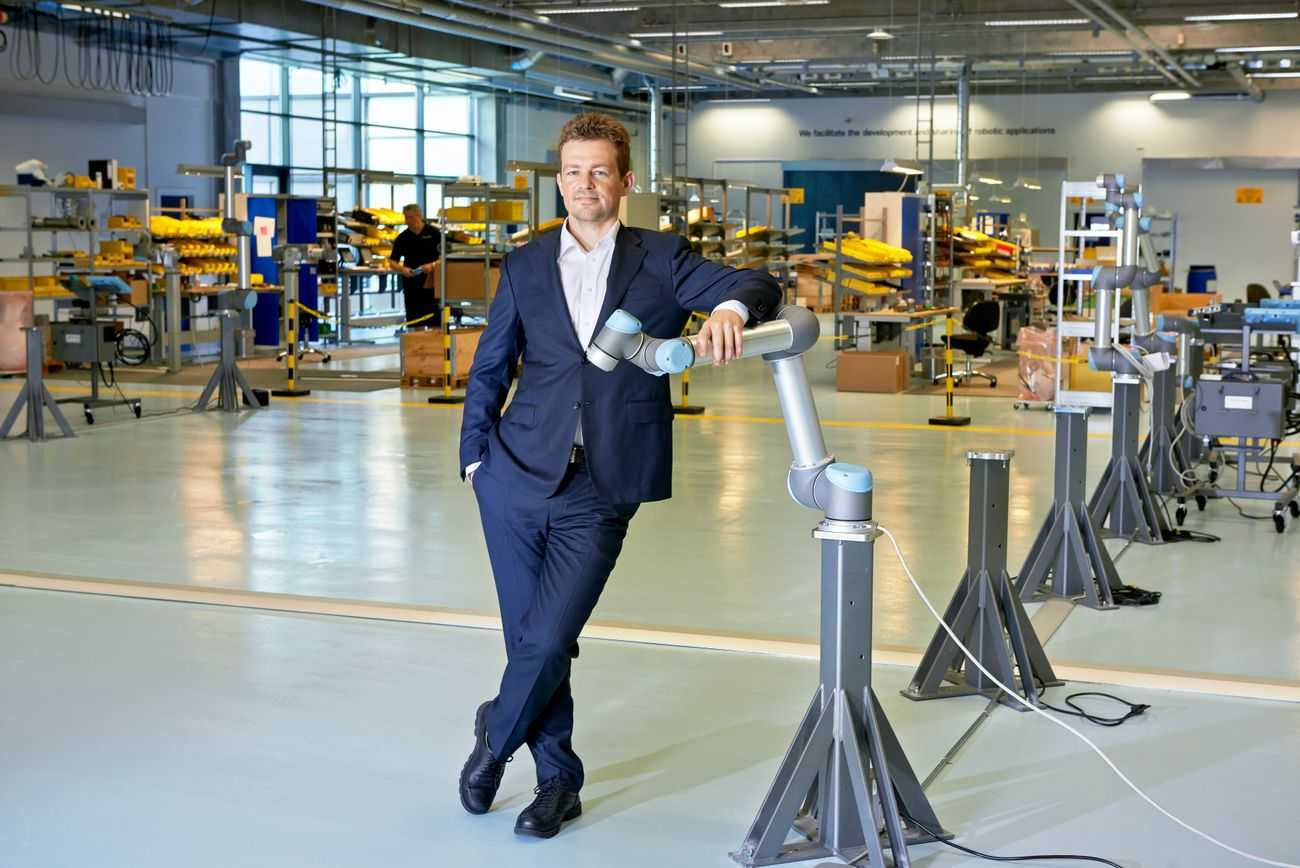Esben Østergaard is the founder of Universal Robots, a startup that wants to automate industry. Speaking to IMPO, he makes the point that robots can be our collaborators rather than our rivals:

“Imagine if you had to put in screws on a table all day long with a traditional screwdriver, then someone came along with an electric screwdriver. You could say it is taking away work from you but no one would see it that way.”

Of course, this is the view of a man with vested interests. But it’s already possible to see human-robot collaborations in action.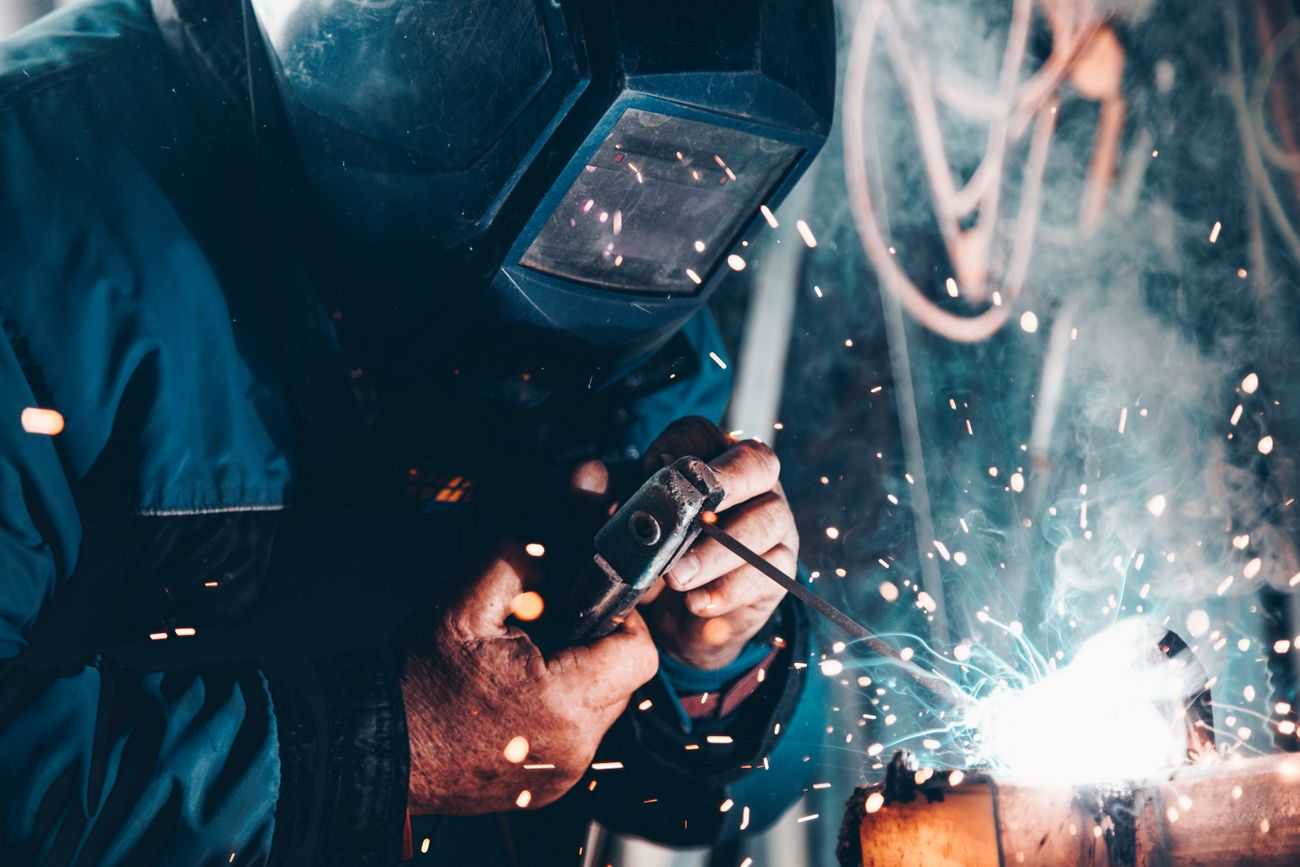Just last year, Adidas installed three industrial knitting machines in one of its German stores. Whenever customers wanted something unique, staff could simply create a new garment on the spot.

Okay, so this shopping-mall factory could only produce 10 garments each day. And yes, humans had to operate the machines. But it’s easy to see where this kind of automation could take us in the future.You never know — maybe one day your new iPhone will be made in the Apple Store while you wait.

What do you think the future of manufacturing will look like? Tell us in the comments!

#### Meet Mark Myerson

Mark is best known for writing about apps, but he also loves the tactile, hardware side of technology. Being a professional photographer, he's pretty handy with a camera, and he's a self-confessed tweetaholic.

Click or drag your image here (Maximum Size 4MB, Accepted formats JPG, PNG, GIF).
• 😂
• ❤️
• ♥️
• 😍
• 😭
• 😊
• 😒
• 😘
• 💕
• ☺️
• 😩
• 👌
• 😔
• 😏
• 😁
• 😉
• 👍
• 🙏
• 😌
• 🎶
• 😳
• 🙌
• 🙈
• 😢
• 😎
• ✌️
• 👀
• 😅
• 😴
• 😄
• 💜
• 💔
• 💯
• 😑
• 💖
• 💙
• 😕
• 💁
• 😜
• 😞
• 😋
• 😐
• 😪
• 👏
• 💘
• 💗
• 💞
• ⬅️
• 🙊
• 💋
• 👉
• 🌸
• 😱
• 🔥
• 😡
• 😃
• 🎉
• 👊
• 😫
• 📷
• 🌹
• 😝
• 💪
• 💀
• ☀️
• 💛
• 😤
• 🌚
• 😆
• 😓
• 👈
• ✔️
• 😻
• 😀
• 😷
• 💚
• 👋
• 😣
• 💓
• ▶️
• ◀️
• ↪️
• ↩️
• 👑
• 😚
• 😛
• 😥
• 😇
• 🎧
• 😖
• 😠
• 😬
• 🌟
• 🔫
• 🙋
• 👎
• 💃
• 🎵
• 😶
• 💫
• 👇
• 🔴
• 🙅
• 💥
• 💭
• 👅
• 💩
• 😰
• 💎
• 🙆
• 🍕
• 😹
• 🌞
• 🍃
• 💦
• 🐧
• 💤
• 🚶
• ✈️
• 🎈
• 🎀
• ☑️
• 😟
• 🔞
• 😨
• 🍀
• 🌺
• 🎤
• 👐
• 👻
• 🌴
• ‼️
• 💅
• 👽
• 🙇
• 👼
• 👯
• ❄️
• ☝️
• 😙
• 🌈
• 🌙
• 💟
• 💝
• 🎁
• 🍻
• 😧
• 🌍
• 🎥
• ♣️
• ✖️
• 🏃
• 🌻
• 🌎
• 💐
• 🐶
• 💰
• 🌿
• 👫
• 🍂
• 🌷
• 🎂
• 🐱
• 😵
• 👆
• 😮
• 😯
• 🏀
• 🎄
• 💍
• 🌝
• 😲
• 👭
• 💸
• 😿
• 🙉
• 💨
• 🌵
• ♨️
• ☎️
• 🍁
• 👸
• 💆
• 💌
• 🏆
• 🙍
• 🎊
• 🌼
• 🔪
• 👄
• 🍟
• 🍩
• 😦
• 🌊
• 💣
• 🆗
• 🌀
• 🚀
• 💏
• 🍭
• 🎬
• 🐷
• 😈
• 👿
• 🐝
• 😽
• 💢
• 🎼
• 🎅
• 🌏
• 🏈
• 🎸
• ♦️
• 🐼
• 💬
• 🍓
• 😼
• 🍌
• 🍉
• 😸
• ♠️
• 🔝
• 🍆
• 🔮
• 🍴
• 📲
• 📱
• ⚠️
• 🙀
• 🔸
• 👶
• 🐾
• 👣
• 🍺
• 🍷
• 📹
• 🐰
• 🍹
• 🚬
• 👾
• 🍑
• 🐍
• 🐢
• 🍒
• 😗
• 🐸
• 🌌
• 🚨
• 🐣
• 📕
• 🍬
• 🍔
• 🐻
• 🐯
• 🚗
• 🍦
• 🍍
• 🌾
• 💉
• 🚮
• 🍫
• ▪️
• 📺
• 💊
• 🐙
• 🎃
• 🍇
• 😺
• 💿
• 🍸
• 🍰
• 🎮
• ™️
• ⬇️
• 🚫
• 💄
• 🐳
• 📝
• ®️
• 🍪
• 🐬
• 🔊
• 👨
• 🐥
• 🐒
• 📚
• 👹
• 💂
• 📢
• ✂️
• 👧
• 🎓
• ⚾️
• 🚦
• 👩
• 🎆
• 🌠
• 🆘
• 🍄
• 😾
• 🛅
• 👠
• 🎯
• 🏊
• 🔑
• 👙
• 👪
• 🐘
• 💧
• 🌱
• 🍎
• 🆒
• 📞
• 💵
• 🏡
• 📖
• 💇
• 💻
• 💡
• 🔙
• 👦
• 🔐
• 🙎
• 🍊
• ↔️
• 🌅
• 🍗
• 🔵
• 🚘
• 🍧
• 🐦
• 🌛
• 👓
• 🐐
• 🌃
• 👵
• 🌑
• 👬
• 🛃
• 🐠
• 🏠
• 🔃
• 🌜
• 📍
• 🌕
• 👟
• 🍋
• 🍼
• 🎨
• ✉️
• 🍝
• 🎐
• 🍥
• 🌲
• 🆙
• ⬆️
• ↗️
• ↘️
• ↙️
• 🎭
• 👃
• 🐽
• 🐟
• 👳
• 🐨
• 👂
• ✳️
• 🔹
• 🚿
• 🐛
• 🍜
• 🎩
• 👰
• 🏁
• 🐴
• 🐵
• 🚼
• 🆕
• 🆓
• 🎇
• 🌽
• 🎾
• 🔋
• 🐺
• 🗿
• 🐮
• 📣
• 👴
• 👗
• 🔗
• 🐔
• 🍳
• 🐋
• 🌳
• 🍱
• 📌
• 🔜
• 🔁
• 🐉
• 🐹
• 🏄
• 🐭
• 🌒
• 🚙
• 🅰️
• ⁉️
• 🔌
• 🌓
• 🔱
• 🍞
• 👮
• 🍵
• 🎣
• 🌔
• 🚲
• 👤
• 🍚
• 📻
• 🐤
• ⤵️
• 🌘
• 🌗
• 🔘
• 🐑
• 👱
• 🌖
• 🔒
• 🍏
• 👺
• 🚩
• 🔄
• 🐎
• 🍤
• 🌄
• 🌋
• 🐓
• 📥
• 💒
• 🍣
• 🍨
• 🍅
• 🐇
• ✴️
• 🔺
• 🔆
• 👲
• 🏪
• 👥
• 🐞
• 🔻
• ⤴️
• 📛
• 🛀
• 🐊
• 🌰
• 🐕
• 🐈
• 🔨
• 🍖
• 🐚
• ❇️
• 🅱️
• Ⓜ️
• 🐩
• 🍲
• 👖
• 🍯
• 🎹
• 🔓
• 🗽
• 💲
• 🏂
• 💮
• 👔
• 💠
• 🚺
• 🐜
• 🌇
• 🅾️
• 🐲
• 🐌
• 📀
• 👕
• 🎲
• 🎎
• 🎱
• 🚌
• 🍮
• 🎌
• 〽️
• 🐫
• 🍛
• 🚂
• 🏥
• 🔷
• 🎋
• 🔔
• 🍐
• 🔶
• 🌐
• 🚪
• 🕕
• 🚔
• 📩
• 🌂
• 🎷
• 🚴
• 🍡
• 🏢
• 🚣
• 👒
• 👞
• 🏩
• 🗻
• 🐪
• 👜
• 🎺
• 🏫
• 🐄
• 🌆
• 👷
• 🚽
• 🐖
• 🔰
• 🎻
• 🔛
• 💳
• 🆔
• 🎡
• 🎳
• 💈
• 👛
• 🎢
• 🐀
• 📅
• 🏉
• 🐏
• 🔼
• 🔲
• 📴
• 🗼
• 👘
• 🚢
• 🔎
• 🔍
• 🚒
• 🕦
• 🚓
• 🃏
• 🌉
• 📦
• 🚖
• 📆
• 🏇
• 🐅
• 👢
• 🚑
• 🔳
• 🐗
• 🎒
• 💷
• 🐂
• 🍙
• 🆚
• 🔚
• 🅿️
• 👡
• 💺
• 🚕
• 💼
• 📰
• 🎪
• 🔯
• 🚹
• 🏰
• 🔦
• 🌁
• 🎍
• 🎫
• 🚁
• 💽
• 🚍
• 🍈
• 🏤
• 🔟
• 📓
• 🔕
• 🍢
• 🎏
• 🎠
• 🐡
• 📈
• 🍠
• 🎿
• 🕛
• 📶
• 🚧
• #
• 📡
• 💶
• 👚
• 📒
• 🐆
• 🔅
• 🕒
• 🏬
• 🚚
• 🍶
• 🚃
• 🚤
• 📼
• 🕐
• 🐃
• 🔽
• 💴
• 🔇
• 🎽
• 🕑
• 📎
• 🏧
• 🎦
• 🔭
• 🎑
• 📘
• ◻️
• 📮
• 📧
• 🐁
• 🚄
• 🔩
• 🆖
• 🏨
• 🚾
• 🏮
• 🔂
• 📬
• 📉
• 📗
• 🚜
• 🚇
• 📋
• 📵
• 🕓
• 🚭
• 🎰
• 🕔
• 🛁
• 📜
• 🚉
• 🍘
• 🏦
• 🔧
• 🈯️
• 🚛
• 📄
• 📊
• 🚷
• 📳
• 🕙
• 🕘
• 🚅
• 🚐
• 🚊
• 🕗
• 🈳
• 🚥
• 🚵
• 🔬
• 🏯
• 🔖
• 📑
• 👝
• 🆎
• 📃
• 🎴
• 🕚
• 📠
• 🕖
• 💱
• 🔉
• 💹
• 🆑
• 💾
• 🏣
• 🔈
• 🗾
• 🈺
• 🀄
• 📨
• 📙
• 🚻
• 🈚️
• 🈶
• 📐
• 🚋
• 🈸
• 🚎
• 🈷
• 🔢
• 📔
• 🈲
• 🈵
• 📯
• 🏭
• 🚸
• 🚆
• 📏
• 📟
• 🉑
• 🈴
• 🔏
• 🕜
• 🈂️
• 📤
• 🔀
• 📫
• 🚈
• 🕤
• 🚏
• 📂
• 📁
• 🚰
• 📇
• 🕝
• 🚝
• 🕧
• 🕥
• 🔤
• 📪
• 🕟
• 🚞
• 🚯
• 🕞
• 🕢
• 🕠
• 🔠
• 📭
• 🔣
• 🚡
• 🕣
• 🕡
• 🔡
• 🚠
• 🈁
• 🛂
• 🚱
• 🚟
• 🛄
• 🚳
• 🏳‍🌈
• 🕵
• 🤗
• 🤖
• 🤕
• 🤔
• 🤓
• 🤒
• 🤑
• 🤐
• 🙄
• 🙃
• 🙂
• 🙁
• 🤘
• 🖖
• 🖕
• 🖐
• 🕶
• 👁‍🗨
• 👁
• 🏋
• 🕴
• 🏌
• ❣️
• 🛢
• 🛡
• 🛠
• 🛏
• 🛎
• 🛍
• 🛌
• 🛋
• 🗳
• 🗡
• 🗞
• 🗝
• 🗜
• 🗓
• 🗒
• 🗑
• 🗄
• 🗃
• 🗂
• 🖼
• 🖲
• 🖱
• 🖨
• 🖥
• 🖍
• 🖌
• 🖋
• 🖊
• 🖇
• 🕹
• 🕳
• 🕰
• 🕯
• 📿
• 📽
• 📸
• 🏺
• 🏷
• 🏴
• 🏳
• 🎞
• 🎛
• 🎚
• 🎙
• 🌡
• 🛳
• 🛰
• 🛬
• 🛫
• 🛩
• 🛥
• 🛤
• 🛣
• 🗺
• 🕍
• 🕌
• 🕋
• 🏟
• 🏞
• 🏝
• 🏜
• 🏛
• 🏚
• 🏙
• 🏘
• 🏗
• 🏖
• 🏕
• 🏔
• 🏎
• 🏍
• 🏹
• 🏸
• 🏵
• 🏓
• 🏒
• 🏑
• 🏐
• 🏏
• 🏅
• 🎟
• 🎗
• 🎖
• 🧀
• 🍿
• 🍾
• 🍽
• 🌶
• 🌯
• 🌮
• 🌭
• ☃️
• ☂️
• 🦄
• 🦃
• 🦂
• 🦁
• 🦀
• 🕸
• 🕷
• 🕊
• 🐿
• 🌬
• 🌫
• 🌪
• 🌩
• 🌨
• 🌧
• 🌦
• 🌥
• 🌤
• 🗣
• 🖤
• 🗨
• 🥚
• 🛑
• 🥁